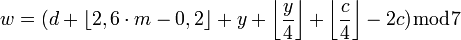# crazy maths quiz.

## it is a quiz which is very hard to answer.and it involves 4 options.it is created to let people know the level in which they are in mathematics.

0.12,4
o.23,2
0.12,3
0.12,2

### x^x+y^y=31,x+y=5.find x and y.

Select the one correct answers
3 and 2
4 and 2
5 and 3
0 and 2

a^x.lnx
a^x
a^x/(lnx)
lnx

duododododcagon
heligon
paragon
2000-gon

1
5
6
10

n!=m^2
n!+1=m^2
m!=n-2!
m^2=n!-16

sexagon
vigesimal
sexagesimal
hexagesimal

root 5
sin30
pi
log5